# Simple muscle-lever systems are not so simple: The need for dynamic analyses to predict lever mechanics that maximize speed

## Cite this dataset

Cox, Suzanne (2021). Simple muscle-lever systems are not so simple: The need for dynamic analyses to predict lever mechanics that maximize speed [Dataset]. Dryad. https://doi.org/10.5061/dryad.zpc866t71

## Abstract

Here we argue that quasi-static analyses are insufficient to predict the speed of an organism from its skeletal mechanics alone (i.e. lever arm mechanics). Using a musculoskeletal numerical model we specifically demonstrate that 1) a single lever morphology can produce a range of output velocities, and 2) a single output velocity can be produced by a drastically different set of lever morphologies. These two sets of simulations quantitatively demonstrate that it is incorrect to assume a one-to-one relationship between lever arm morphology and organism maximum velocity. We then use a statistical analysis to quantify what parameters are determining output velocity, and find that muscle physiology, geometry, and limb mass are all extremely important. Lastly we argue that the functional output of a simple lever is dependent on the dynamic interaction of two opposing factors: those decreasing velocity at low mechanical advantage (low torque and muscle work) and those decreasing velocity at high mechanical advantage (muscle force-velocity effects). These dynamic effects are not accounted for in static analyses and are inconsistent with a force-velocity tradeoff in lever systems. Therefore, we advocate for a dynamic, integrative approach that takes these factors into account when analyzing changes in skeletal levers.

## Methods

We built a computational model of a simple lever system using OpenSim V. 4.0, scripting in MATLAB (MathWorks; Natick, MA).  Our model was composed of an output lever (length 0.12 m, width and height 0.04 m) that pivoted around a pin joint, a stand to connect the pin-joint to the world and a muscle that originated on the stand, wrapped around a wrap cylinder and attached to the lever (figure 1). These values were chosen to approximate the tarsometatarsus of the guinea fowl since detailed experimental data was available.   The diameter of the wrapping surface defined the input lever or moment arm of the muscle acting at this joint and allowed the muscle to change length and rotate the lever without altering the line of action. The muscle implemented was a Millard2012EquilibriumMuscle muscle model using default length-tension and force-velocity  values with a non-compliant tendon and a fixed pennation angle of zero.   The lever had the inertial properties of a rectangular rod defined by its volume with a density of water (997 kg m-3).  Gravity was not included in the model. To simulate the system driving a mass, we welded a mass on the end of the lever in the shape of a sphere with the density of water. We constrained the lever’s range of motion to 150 degrees with a joint limiting force stiffness of 20, damping of 5 and transition range of 2.5 degrees.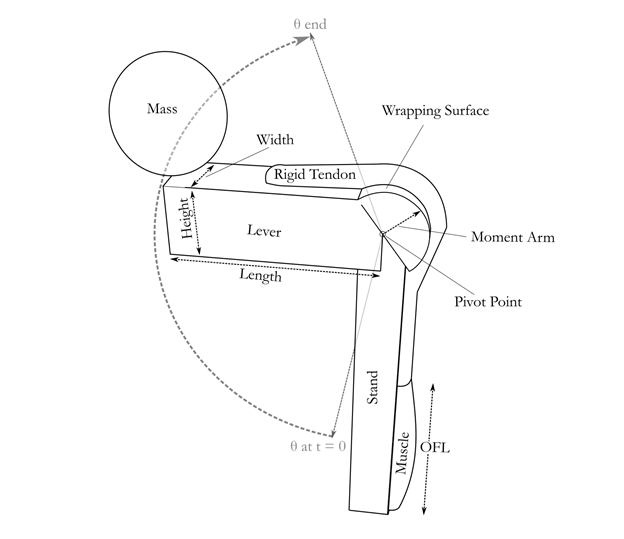We generated 3600 modifications of this model with different muscle optimal fiber lengths (OFL:(0.01:0.01:0.1 m), moment arms (0.0005:0.0005:0.02 m), starting normalized muscle lengths (0.8,1.1,1.4) and driven masses (0.01 ,0.5, 2 kg). Since muscle-tendon unit length changed with moment arm, we adjusted the tendon slack length for each model such that the equilibrium normalized fiber length for the passive muscle was within 0.01 units of a fixed starting fiber length. We held the volume of the muscle constant across changes in optimal fiber length.  Since the maximum isometric force of a muscle is a function of its cross-sectional area, iso-volumetric muscles imply that the force capacity, Fmax, is inversely related to optimal fiber length, OFL, such that

FmaxOFL = M Tsρ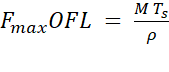,

where M is muscle mass, Ts is the specific tension of the muscle and ρ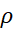is muscle density. The muscle was modeled on the sum of the lateral and medial gastrocnemius muscles of the guinea fowl, with a muscle mass of 17g, a specific tension of 3e5 N/m2 and a muscle density of 1060 kg/m3.  For each modified model, we simulated motion resulting from 100% activation of the muscle model across the full range of motion of the joint in 0.0005 second time steps.

At each time step we extracted the joint angle, angular velocity and acceleration of the lever as well as the muscle active-fiber force, fiber length, and fiber velocity. We trimmed this data to the time range over which the velocity of the mass was increasing. To distinguish the torque required to accelerate the lever from that available to accelerate the mass, we calculated them separately.  The force acting to accelerate the mass was calculated from the torque accelerating the mass, τm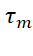, and the distance from the center of the mass to the pivot, d.

Fm=τmd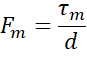The torque acting to accelerate the mass was calculated from the acceleration of the mass and its moment of inertia rotating around the pivot, calculated with the parallel axis theorem;

τm = 25 mr2+md2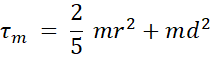where d is the distance from the center of mass to the pivot point,h r is the diameter of the added mass, and m is the mass of the added mass.  The torque acting to accelerate the lever was similarly calculated.

Additionally, impulse of the muscle and impulse applied to the mass were calculated as the integral of the active fiber force of the muscle and the force applied to the mass, respectively.

## Usage notes

The code used to generate the data files, the resulting data, and a read-me explaining the variables in the data file.

## Funding

Royal Society, Award: UF120507

UK MRC, Award: MR/T046619/1

United States Army Research Office, Award: W911NF-15-038

UK MRC, Award: MR/T046619/1